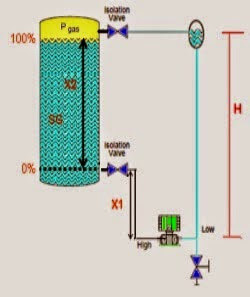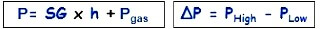# Closed tank DP Level Transmitter with wet leg elevation zero remote mount Calibration

The DP level transmitter installed below HP tapping point with wet leg.Schematic Diagram of a Closed tank DP Level Transmitter with wet leg elevation zero remote mount CalibrationClosed tank DP Level Transmitter with wet leg elevation zero remote mount CalibrationFormulas:

Min range = (SG x X1) – (SG x H)

Max range = [(SGxX1) + (SGxX2)] – (SGxH)

Example:

If the height is 1 meter an X1, X2 is 3 meters and a height H is 4.5 meters, in the tank there is cairin which has a density of 1 g / cm3, what is the range of calibration on the transmitter?

SG = 1

Min range = P High – P Low

= (SG x X1) – (SG x H)
= (1 x 1) – (1 x 4.5)
= 1 -4.5
= – 3.5 mH 2 O

Max range = P High – P Low

= [(SGxX1) + (SGxX2)] – (SGxH)
= [(1×1) + (1×2) – (1×4,5)
= 4 to 4.5
= -0.5 MH 2 O

The transmitter Range = -3.5 to -0.5 mH 2 O

Be the first to get exclusive content straight to your email.
We promise not to spam you. You can unsubscribe at any time.

### 4 thoughts on “Closed tank DP Level Transmitter with wet leg elevation zero remote mount Calibration”

1.Easy to learn.. but all students needs to calculate the range by themselves.. beacause one printing mistake is there.

•2.3.